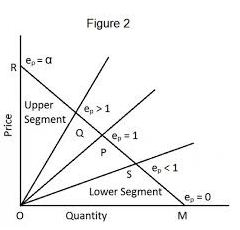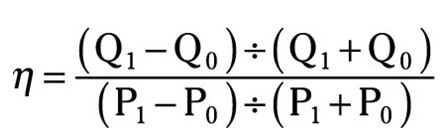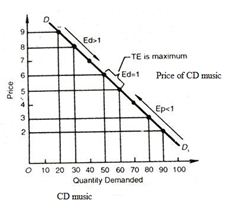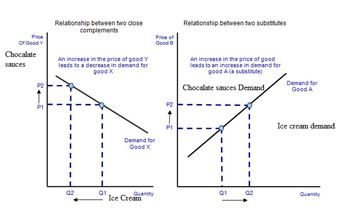# Economics and Quantitative Analysis Paper Editing Services## Introduction

Demand and price of the products in the market both are the valuable factors which is dependent upon various determinates in the given market segment. In this report demand elasticity and cross elasticity of demand has been taken into consideration.

### Body context

#### Question 1 Midpoint method for calculating the price elasticity of demand?

Price elasticity of the demand- It is the method which is used to identify the responsiveness of the demand changes due to changes in the price.

In the increasing trends of the economic changes, there are used several methods to identify the midpoint of the price elasticity of demand.

Some methods are as follows:-

1. Percentage method:

It is the very valuable method which is used to evaluate the percentage change in the quantity of the goods due to a percentage change in the price of the products. It is measured by the changes in the percentage of the quantity of the product in the given project.

Elasticity of demand(Ed) = % change in the quantity demanded / % change in the price.2. Geometric method:-

It is a method which is used to evaluate the measure of elasticity of the demand curve. This method is used to identify the infinity changes in the price and demand for the product. For calculating the Ed at any particular point lower portion of the curve is used to dividing the upper portion of the curve from the same point.

Formula, which is used to evaluate the price elasticity of demand:-Where:-

η   = Represent the price elasticity of products.

Q0 = it represents the initial quantity of the products offered in the market.

Q1 = provides new quantity of the products.

The main advantages of midpoint method as follows:-

There are various benefits of using this method. Basically, it assists in determining the actual price of the products in a determined manner. (De Grange, et., Al., 2013).

### Question 2. Key determinants of the price elasticity of demand for a product?

Price elasticity of demand for a product is depending upon the how much change in the price arises due to changes in the demand for the product. Basically, this price of demand is totally depending on the following factors as follows.

Availability  of substitute goods:

When several close substitutes are available then greater the elasticity of demand for a product exists, through which consumer can easily switch from one good to another (He & Zhao, 2016).

Products that consume the high portion of the client’s revenue tend to have a greater elasticity of the demand in the market comparatively.

Degree of necessity:

There are consumers will attempt to purchase necessary products regardless of the price, e.g. medicine.

Duration of price change:

For non-durable goods, elasticity tends to be greater over long-run than in the short-run. In short-run it becomes difficult for consumers to find out the substitute in response to a price change. But, in a long time consumers can adjust their behaviour.

Brand loyalty:

It creates image towards the product in the mind of consumers. It helps to increase the price of the product and provide a clear level in the mind of consumers (Esteves &Reggiani,  2014).

There are four important determinants of price elasticity of demand:

Availability of substitute goods
Degree of required goods
Duration or time span of the subjective product

Price elasticity of the demand- It is the Arc curve which measures the relationship between price and demand of the products. It is observed that this price elasticity measure the reciprocate behaviour of the quantity demanded or supplied of goods or services due to changes in the price in determined approach. Elasticity could be described as elastic, unit elastic and elastic.

Elastic = > 1= % change in the quantity/ % change in the price

Unitary= 1 = % change in the quantity/ % change in the price

Inelastic=< 1=% change in the quantity/ % change in the price

In this question, it is found that Universal music has to make changes in its price in order to increase the demand for CD music in the market. In the price elasticity, it could be seen that Universal music has proposed the plan to boost the quantity of the CD music by 30 % with the view to reduce the price of the music in the market.

Price decision- By analyzing all the required factors in the universal music has proposed plan to decrease the price of the CD music. however, it could be seen that by reducing the price of the CD music will surely result into the increment in the demand but the use of internet and downloading has been showcasing high impact on the demand of the CD music sell in the market. With the computation, it is found that price elasticity of the demand is .90 and percentage change in the price is 33 %.### Question- 4 I Tunes raised

Price elasticity of demand- It is the demand curve which represents the changes in the demand for the products due to changes in the price of the same products.

In this question, it has been given that I tunes have raised its price of 33 songs from .99 to \$ 1.29 per downloads which results into fell into the demand of the I tunes songs demand in the market by 35 % (Andreyeva, et. Al.,  2010).

Price elasticity of demand=% changes in the price/ % change in the quantity

0.30303/ .35= 0.8

By making computation it could be found that Price elasticity of demand of I tunes is .8.

### Question-5 Chocolate sauces

In this case study, it is found that price of chocolate sauces is decreased and that results in the increase in the demand. However, the price elasticity of demand for chocolate sauces could be shown with the help of formula shown below.Price elasticity demand of the sauces= % changes in the price/ % change in the quantity

5% /10% = .5

By evaluating this data it could be said that price elasticity demand of sauces is .5.

Cross elasticity demand for Chocolate-This elasticity demand describe the relationship between changes in the quantity demand for ice-cream due to change in the price of Chocolate sauces.

Cross elasticity demand of the chocolate= % changes in the quantity of sauces / % change in the quantity of Ice cream

10%15%= 0.33

#### Concussion

In this report, it is found that demand and price of the products in the market have the negative relation. Therefore if one organization wants to increase the demand for the product then there is need to decrease the price of same products if other things remain the same.

#### References

Andreyeva, T., Long, M.W. & Brownell, K.D. 2010, "The Impact of Food Prices on Consumption: A Systematic Review of Research on the Price Elasticity of Demand for Food", American Journal of Public Health, vol. 100, no. 2, pp. 216-222.

Andreyeva, T., Long, M.W. & Brownell, K.D. 2010, "The Impact of Food Prices on Consumption: A Systematic Review of Research on the Price Elasticity of Demand for Food", American Journal of Public Health, vol. 100, no. 2, pp. 216-222.

De Grange, L., Gonzalez, F., Munoz, J.C. &Troncoso, R. 2013, "Aggregate estimation of the price elasticity of demand for public transport in integrated fare systems: The case of Transantiago", Transport Policy, vol. 29, pp. 178.

Esteves, R. &Reggiani, C. 2014, "Elasticity of demand and behaviour-based price discrimination", International Journal of Industrial Organization, vol. 32, pp. 46-56.

He, Y. & Zhao, X. 2016, "Contracts and coordination: Supply chains with uncertain demand and supply", Naval Research Logistics (NRL), vol. 63, no. 4, pp. 305-319.